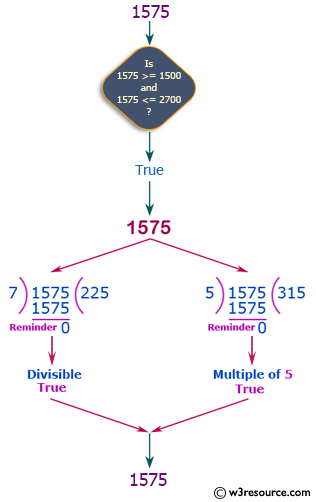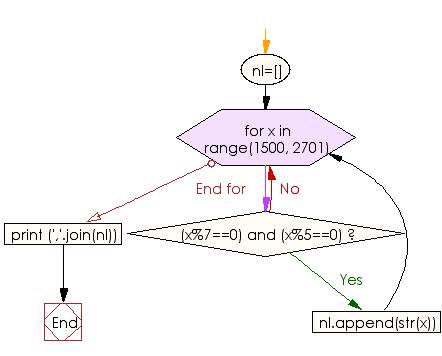﻿ Python Exercise: Find numbers which are divisible by 7 and multiple of 5 between a range - w3resource# Python Exercise: Find numbers which are divisible by 7 and multiple of 5 between a range

## Python Conditional: Exercise-1 with Solution

Write a Python program to find those numbers which are divisible by 7 and multiple of 5, between 1500 and 2700 (both included).

Pictorial Presentation:Sample Solution:

Python Code:

``````nl=[]
for x in range(1500, 2701):
if (x%7==0) and (x%5==0):
nl.append(str(x))
print (','.join(nl))
```
```

Sample Output:

```1505,1540,1575,1610,1645,1680,1715,1750,1785,1820,1855,1890,1925,1960,1995,2030,2065,2100,2135,2170,2205,2240,
2275,2310,2345,2380,2415,2450,2485,2520,2555,2590,2625,2660,2695
```

Flowchart:## Visualize Python code execution:

The following tool visualize what the computer is doing step-by-step as it executes the said program:

Python Code Editor:

Have another way to solve this solution? Contribute your code (and comments) through Disqus.

What is the difficulty level of this exercise?

Test your Python skills with w3resource's quiz

﻿

## Python: Tips of the Day

Returns a list with n elements removed from the beginning

Example:

```def tips_take(itr, n = 1):
return itr[:n]
print(tips_take([1, 2, 3], 5))
print(tips_take([1, 2, 3], 0))
```

Output:

```[1, 2, 3]
[]
```